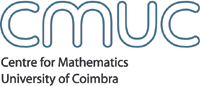AnalysisMembersSeminarsEventsPublicationsdetails Title A sensitivity and adjoint calculus for the optimal control of flows with shocks AbstractOptimal control or design problems for hyperbolic conservation laws arise for example in the design of ducts and airfoils or in the identification of friction parameters in shallow water flows after neglecting viscous effects. A key point for an efficient solution of the resulting optimization problems is the question of (Frechet-)differentiability of the objective functional. It is well known that entropy solution of conservation laws develop in general discontinuities (shocks) after a finite time. Since a variation of the control leads to a variation of the shock locations, the control-to-state mapping is a priori at best differentiable for a very weak topology on the state space. This causes difficulties for the analysis as well as for an efficient numerical solution of the considered control problems. We present a sensitivity calculus in L^1 for scalar conservation laws with source terms that is based on a first order approximation by nonlinear shift-variations. This sensitivity calculus implies the Frechet-differentiability of objective functionals of tracking type. Furthermore, we derive an adjoint-based gradient representation for tracking type functionals. A key ingredient is an adjoint formula for shock sensitivities and a careful analysis of the adjoint equation. The adjoint state has to be defined as reversible solution of the in general not uniquely solvable adjoint equation. We discuss important stability properties of reversible solutions which can in turn be used to define measure solutions of the sensitivity equations by a duality relation. Moreover, we discuss which discretization schemes of the state equation lead to convergent numerical adjoints and sensitivities. For this schemes, the discrete gradients converge to the gradient of the continuous problem. The discrete gradient can for example be computed by using automatic differentiation tools where the forward mode yields a sensitivity-based, the backward mode an adjoint-based gradient computation. We show by numerical results for the optimal control of flows with shocks in 1- and 2-D, in particular the optimal design of an airfoil, that the consideration of our analytical results leads to efficient optimization methods. Speaker(s) Stefan Ulbrich, Technische Universitat Munchen, Germany Date September 15, 2000Time Room Room 5.5 CMUC Apartado 3008, 3001 - 454 Coimbra, Portugal T:+351 239 791 150 F:+351 239 793 069 cmuc@mat.uc.pt - developed by Flor de Utopia